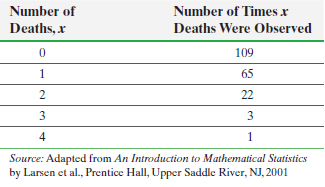×
Get Full Access to Statistics: Informed Decisions Using Data - 5 Edition - Chapter 6.3 - Problem 21
Get Full Access to Statistics: Informed Decisions Using Data - 5 Edition - Chapter 6.3 - Problem 21

×

# ?Prussian Army In 1898, Ladislaus von Bortkiewicz published The Law of Small Numbers, in which he demonstrated the power of the Poisson probability lawISBN: 9780134133539 240

## Solution for problem 21 Chapter 6.3

Statistics: Informed Decisions Using Data | 5th Edition

• Textbook Solutions
• 2901 Step-by-step solutions solved by professors and subject experts
• Get 24/7 help from StudySoup virtual teaching assistantsStatistics: Informed Decisions Using Data | 5th Edition

4 5 1 333 Reviews
28
2
Problem 21

Prussian Army In 1898, Ladislaus von Bortkiewicz published The Law of Small Numbers, in which he demonstrated the power of the Poisson probability law. Before his publication, the law was used exclusively to approximate binomial probabilities. He demonstrated the law’s power, using the number of Prussian cavalry soldiers who were kicked to death by their horses. The Prussian army monitored 10 cavalry corps for 20 years and recorded the number X of annual fatalities because of horse kicks for the 200 observations. The following table shows the data:(a) Compute the proportion of years in which there were 0 deaths, 1 death, 2 deaths, 3 deaths, and 4 deaths.

(b) From the data in the table, what was the mean number of deaths per year?

(c) Use the mean number of deaths per year found in part (b) and the Poisson probability law to determine the theoretical proportion of years that 0 deaths should occur. Repeat this for 1, 2, 3, and 4 deaths.

(d) Compare the observed proportions to the theoretical proportions. Do you think the data can be modeled by the Poisson probability law?

Step-by-Step Solution:

Step 1 of 5) Prussian Army In 1898, Ladislaus von Bortkiewicz published The Law of Small Numbers, in which he demonstrated the power of the Poisson probability law. Before his publication, the law was used exclusively to approximate binomial probabilities. He demonstrated the law’s power, using the number of Prussian cavalry soldiers who were kicked to death by their horses. The Prussian army monitored 10 cavalry corps for 20 years and recorded the number X of annual fatalities because of horse kicks for the 200 observations. The following table shows the data: (a) Compute the proportion of years in which there were 0 deaths, 1 death, 2 deaths, 3 deaths, and 4 deaths. (b) From the data in the table, what was the mean number of deaths per year (c) Use the mean number of deaths per year found in part (b) and the Poisson probability law to determine the theoretical proportion of years that 0 deaths should occur. Repeat this for 1, 2, 3, and 4 deaths. (d) Compare the observed proportions to the theoretical proportions. Do you think the data can be modeled by the Poisson probability law 4UFQ

Step 2 of 2

## Discover and learn what students are asking

Calculus: Early Transcendental Functions : Conics, Parametric Equations, and Polar Coordinates
?In Exercises 1–6, match the equation with the correct graph. [The graphs are labeled (a), (b), (c), (d), (e), and (f).]

Statistics: Informed Decisions Using Data : Data Collection
?In Problems 1–5, provide a definition using your own words. 4. Observational study

Statistics: Informed Decisions Using Data : Data Collection
?Explain the steps in designing an experiment.

Statistics: Informed Decisions Using Data : Discrete Probability Distributions
?Is it appropriate to estimate the average cost of the voyage from the mean family size? Why or why not?

Unlock Textbook Solution